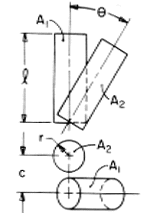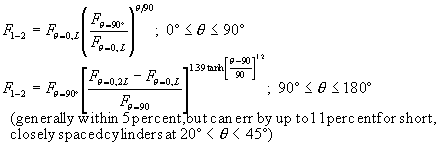# SECTION C Factors From Finite Areas to Finite Areas

## C-105: Cylinders of equal length and radius, rotated around line connecting ends.Definitions:

 Fq=90 from preceding factor in this table (C-104); Fq=0,X for parallel finite cylinders of length X (C-101); L = l/r

Governing Equation:To download a copy of this equation in MS Word 97 Format, click HERE.